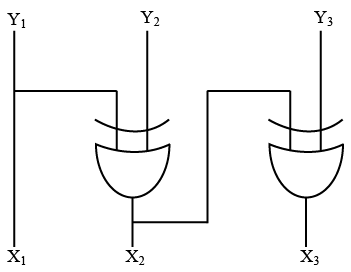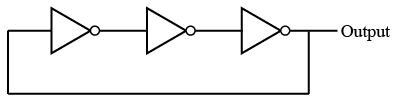# ISRO Scientist or Engineer Computer Science 2016

Instructions

For the following questions answer them individually

Question 11

# The dynamic hazard problem occurs inQuestion 12

# The logic circuit given below converts a binary code $$y_1, y_2, y_3$$ intoQuestion 13

# The circuit shown in the below figure isQuestion 14

# If $$12A7C_{16}=X_{8}$$ then the value of X isQuestion 15

# The Excess-3 Code is also calledQuestion 16

# The simplified Sum of Product form of the following Boolean expression $$(P+\overline{Q}+\overline{R})(P+Q+R)(P+Q+\overline{R})$$Question 17

# Which of the following binary numberis the sameas its 2’s complement?Question 18

# The functional difference between SRflip-flop and JK flip-flop is thatQuestion 19

# Which of the following binary numberis the sameas its 2’s complement?Question 20

# Whatis the output of this C code?#include <stdio.h>void main(){int k = 5;int *p = &k;int **m = &p;printf("%d %d %d\n", k, *p, **m);}OR